# HSPT Math : Negative numbers

## Example Questions

← Previous 1

### Example Question #1 : Negative Numbers

Which of the following operations always gives a negative result?

A negative number multipled by a negative number

A negative number added to a negative number

A negative number divided by a negative number

A negative number subtracted from a negative number

A negative number taken to the power of a positive integer

A negative number added to a negative number

Explanation:

The sum of two negative numbers is always negative, hence, this is the right choice.

As for the other choices:

The product or quotient of two negative numbers is always positive

A negative number taken to the power of a positive integer can be either negative or positive depending on whether the exponent is even or odd.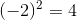, which is positive, and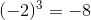, which is negative.

The difference of negative numbers can be either negative, positive, or zero: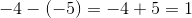, but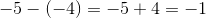### Example Question #2 : Negative Numbers

If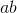is a positive number, and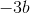is also a positive number, what is a possible value for?Explanation:

Because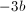is positive,must be negative since the product of two negative numbers is positive.

Because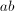is also positive,must also be negative in order to produce a prositive product.

To check you answer, you can try plugging in any negative number for.

### Example Question #3 : Negative Numbers

If x is a negative integer, what else must be a negative integer?

x – x

x – (–x)

x² – x

x – (–x)

Explanation:

By choosing a random negative number, for example: –4, we can input the number into each choice and see if we come out with another negative number.  When we put –4 in for x, we would have –4 – (–(–4)) or –4 – 4, which is –8.  Plugging in the other options gives a positive answer.  You can try other negative numbers, if needed, to confirm this still works.

### Example Question #1 : How To Subtract Negative Numbers

–7 – 7= x

–7 – (–7) = y

what are x and y, respectively

y = 0, x = 14

x = 0, y = 0

x = 14, y = –14

x = –14, y = 14

x = –14, y = 0

x = –14, y = 0

Explanation:

x: –7 – 7= –7 + –7 = –14

y: –7 – (–7) = –7 + 7 = 0

when subtracting a negative number, turn it into an addition problem

### Example Question #5 : Negative Numbers

Solve for: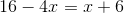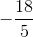Explanation:

Subtractfrom both sides: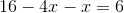, or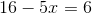Next, subtractfrom both sides: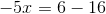, or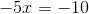Then, divide both sides by: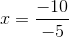Recall that division of a negative by a negative gives you a positive, therefore: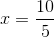or### Example Question #1 : How To Divide Negative Numbers

Solve the following equation: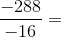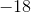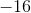Explanation:

The rule for dividing negative numbers is the same as for multiplying negative numbers.

If both numbers are negative, you will get a positive answer.

If either number is positive, and the other is negative, you will get a negative answer.

Therefore: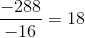### Example Question #1 : Negative Numbers

Choose the answer which best solves the following equation: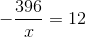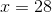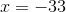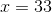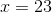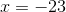Explanation:

To solve, first put the equation in terms of:First multiply the x to both sides.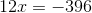Now divide by 12 to solve for x.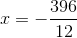Here, because one of the numbers in the equation is positive, and the other is negative, the answer must be a negative number:### Example Question #111 : Arithmetic

Evaluate: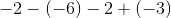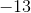Explanation:

To evaluate this, rewrite the expression with the correct signs.

Positive multiplied with a negative sign results in a negative, and a double negative results in a positive sign.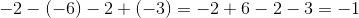### Example Question #3 : How To Add / Subtract / Multiply / Divide Negative Numbers

Subtract: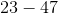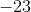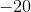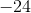Explanation:

It is possible to rewrite the expression as: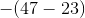Take the negative of the difference of 47 and 23.

The answer is.

### Example Question #4 : How To Add / Subtract / Multiply / Divide Negative Numbers

Solve: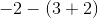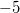Explanation:

Evaluate the inner term inside the parenthesis first.  The expression can then be simplifed to an integer.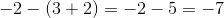← Previous 1

### All HSPT Math Resources